Count cells if starts with a, b or c in range in Excel

In simple words, while working with table values, sometimes we need to check the value in cell whether it starts with A, B or C. Example if we need to find the check of a certain ID value starts with either A, B or C. Criteria inside the formula executed using the operators.

How to solve the problem ?

For this article we will be required to use the COUNTIF function. Now we will make a formula out of the function. Here we are given some values in a range and specific text value as criteria. We need to count the values where the formula checks for the value which starts with either A, B or C.

Generic formula:

= SUM ( COUNTIF ( cell value, { "A*" , "B*" , "C*" } ) ) > 0

Cell value : value where condition is checked.

* : wildcards which find any number of characters within a given cell.

A* : given text or pattern to look for. Use pattern directly in the formula or else if you using cell reference for the pattern use the other formula shown below.

Example:

All of these might be confusing to understand. So, let's test this formula via running it on the example shown below.

Here we have the ID records and we need to find the IDs which starts with either A, B or C.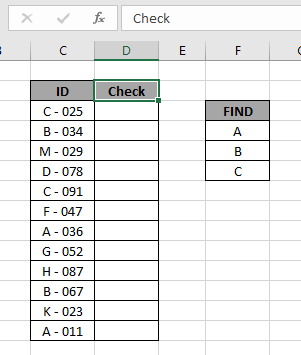Here to find the category IDs which starts with either A , B or C, will be using the * (asterisk) wildcard in the formula. * (asterisk) wildcard finds any number of characters within lookup value.

Use the formula:

= SUM ( COUNTIF ( C3 , { "A*" , "B*" , "C*" } ) ) >0

Explanation:

• COUNTIF function checks the value which matches with either of the pattern stated in the formula
• Criteria is given in using * (asterisk) wildcard to look for value which has any number of characters.
• COUNTIF function returns an array to the SUM function matching either of the values.
• SUM function adds up all the values and checks whether the sum comes out to be greater than 0 or not.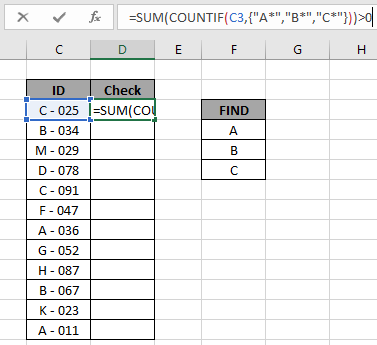Here the range is given as array reference and pattern is given as cell reference. Press Enter to get the count.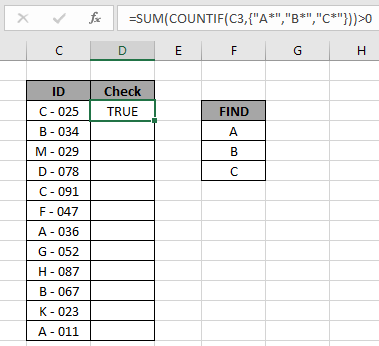As you can see the formula returns TRUE for the value in C3 cell. Now copy the formula in other cells using the drag down option or using the shortcut key Ctrl + D as shown below.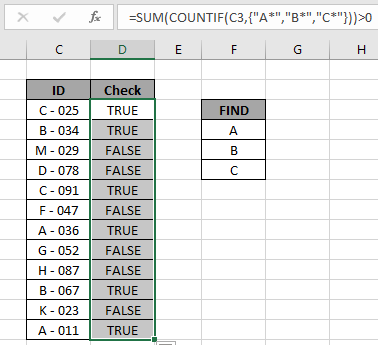As you can see all the TRUE values indicate the cell value starts with either of mentioned pattern and all the FALSE values states there is the cell value starts with neither of mentioned pattern.

Verification:

You can check which values start with a specific pattern in range using the excel filter option. Apply the filter to the ID header and click the arrow button which appears. Follow the steps as shown below.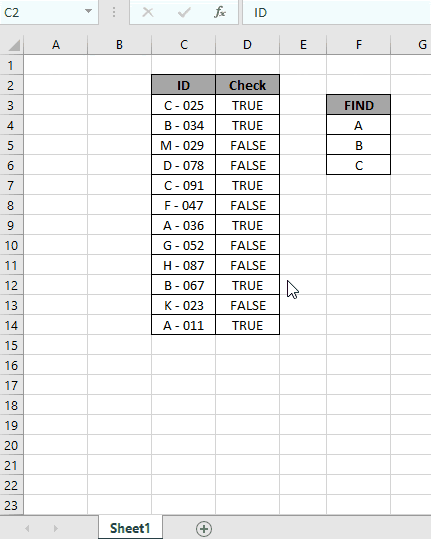Steps:

1. Select the ID header cell. Apply filter using shortcut Ctrl + Shift + L
2. Click the arrow which appeared as a filter option.
3. Deselect (Select All) option.
4. Now search in search box using the * (asterisk) wildcard.
5. Type A* in the search box and select the required values or select the (Select All search result) option as shown in the above gif.
6. Do it for the other two values and get the count of all three which is equal to the result returned by the formula.

As you can see in the above gif all the 6 values which match the given pattern. This also means the formula works fine to get to check the values
Here are some observational notes shown below.

Notes:

1. The formula only works with numbers and text both
2. Operators like equals to ( = ), less than equal to ( <= ), greater than ( > ) or not equals to ( <> ) can be performed within function applied with numbers only.

Hope this article about how to Check values which start with either A , B or C using wildcards in Excel is explanatory. Find more articles on COUNTIF functions here. If you liked our blogs, share it with your fristarts on Facebook. And also you can follow us on Twitter and Facebook. We would love to hear from you, do let us know how we can improve, complement or innovate our work and make it better for you. Write us at info@exceltip.com
Related Articles

How to use the SUMPRODUCT function in Excel: Returns the SUM after multiplication of values in multiple arrays in excel.

COUNTIFS with Dynamic Criteria Range : Count cells depstartent on other cell values in Excel.

COUNTIFS Two Criteria Match : Count cells matching two different criteria on list in excel.

COUNTIFS With OR For Multiple Criteria : Count cells having multiple criteria match using the OR function.

The COUNTIFS Function in Excel : Count cells dependent on other cell values.

How to Use Countif in VBA in Microsoft Excel : Count cells using Visual Basic for Applications code.

How to use wildcards in excel : Count cells matching phrases using the wildcards in excel

Popular Articles

50 Excel Shortcut to Increase Your Productivity : Get faster at your task. These 50 shortcuts will make you work even faster on Excel.

How to use the VLOOKUP Function in Excel : This is one of the most used and popular functions of excel that is used to lookup value from different ranges and sheets.

How to use the COUNTIF function in Excel : Count values with conditions using this amazing function. You don't need to filter your data to count specific values. Countif function is essential to prepare your dashboard.

How to Use SUMIF Function in Excel : This is another dashboard essential function. This helps you sum up values on specific conditions.

Terms and Conditions of use

The applications/code on this site are distributed as is and without warranties or liability. In no event shall the owner of the copyrights, or the authors of the applications/code be liable for any loss of profit, any problems or any damage resulting from the use or evaluation of the applications/code.